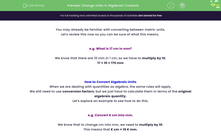# Change Units in Algebraic Contexts

In this worksheet, students will convert algebraic amounts between units by applying one (e.g. kg to g, m to mm, etc.) or two (e.g. m/s to km/h, g/m to kg/km, etc.) conversion factors.Key stage:  KS 4

Year:  GCSE

GCSE Subjects:   Maths

GCSE Boards:   AQA, Eduqas, Pearson Edexcel, OCR,

Popular topics:   Percentages worksheets, Ratio worksheets, Geometry worksheets

Difficulty level:#### Worksheet Overview

You may already be familiar with converting between metric units.

Let's review this now so you can be sure of what this means.

e.g. What is 17 cm in mm?

We know that there are 10 mm in 1 cm, so we have to multiply by 10.

17 × 10 = 170 mm

How to Convert Algebraic Units

When we are dealing with quantities as algebra, the same rules will apply.

We still need to use conversion factors, but we just have to calculate them in terms of the original algebraic quantity.

Let's explore an example to see how to do this.

e.g. Convert K cm into mm.

We know that to change cm into mm, we need to multiply by 10.

This means that K cm = 10 K mm.

Makes sense, doesn't it?

Let's try another, more complex, example now.

e.g. Convert P m/s into km/h.

This is a two-step problem as we have two units of measurement present: distance (m/km) and time (s/h).

1) We know that there are 3600 seconds in 1 hour.

So P metres = 1 second

3600P metres  = 1 hour

2) We know there are 1000 metres in a kilometre.

3600 P metres  = 1 hour

3.6P km = 1 hour

Therefore, P m/s = 3.6P km/hr.

In this activity, you will practise converting algebraic amounts between different units by finding and applying one or more conversion factors.

### What is EdPlace?

We're your National Curriculum aligned online education content provider helping each child succeed in English, maths and science from year 1 to GCSE. With an EdPlace account you’ll be able to track and measure progress, helping each child achieve their best. We build confidence and attainment by personalising each child’s learning at a level that suits them.

Get started